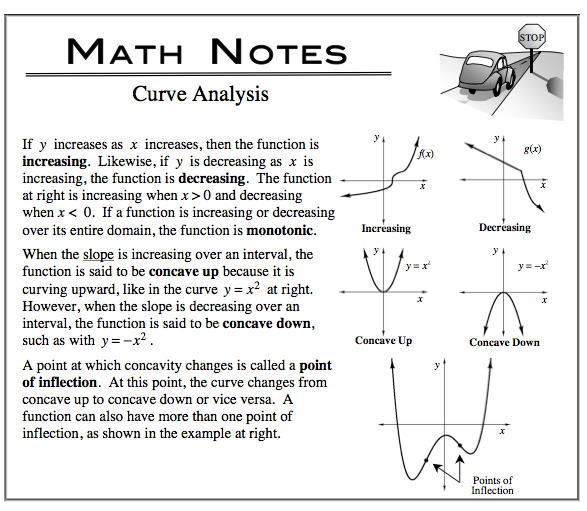### Home > CALC > Chapter 3 > Lesson 3.3.2 > Problem3-109

3-109.

Curves can be labeled with descriptors such as "concave down" and "increasing." On graph paper, graph them and label their respective parts. Use different colors to represent concavity.

1. $f(x) = 2x^{2} + x − 15$

1. $g(x) = x^{3} − 12x − 1$